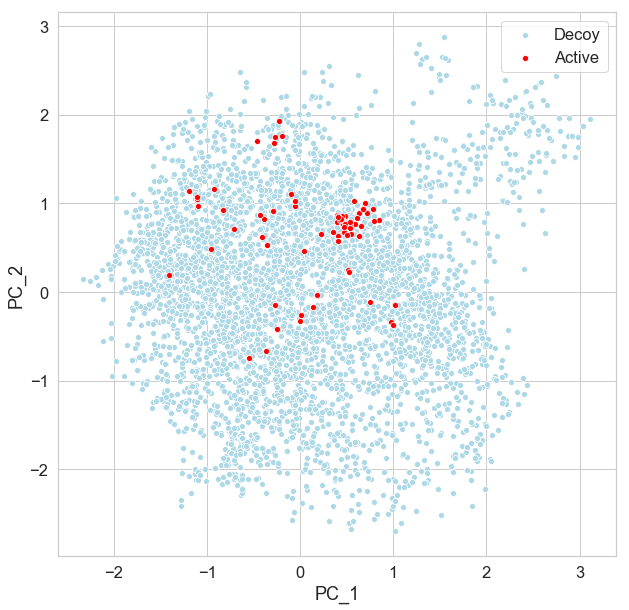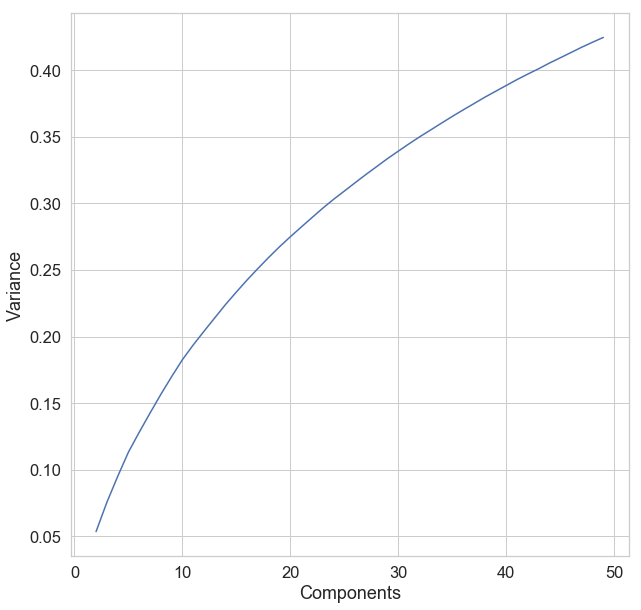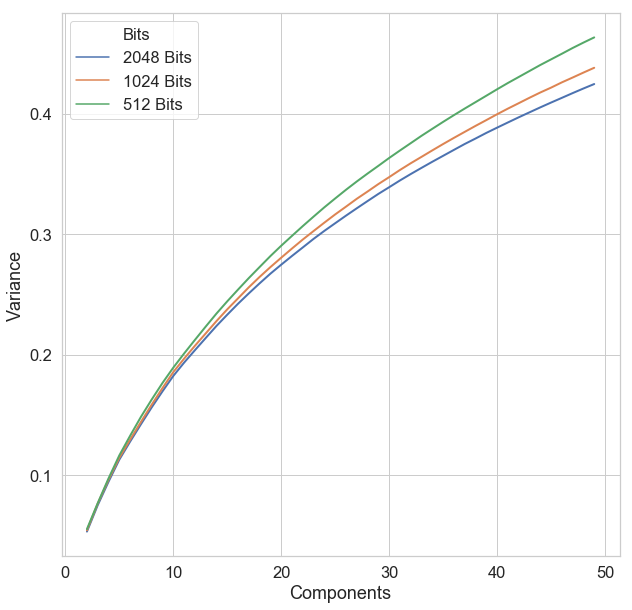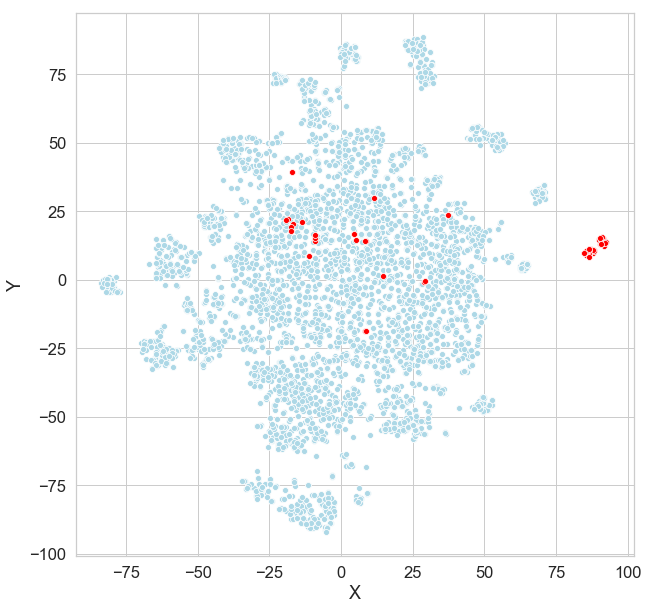### Visualizing Chemical Space

In many cases in Cheminformatics, we need to be able to create a graphical representation of the chemical space covered by a set of molecules.  In this space, similar molecules will be close together and molecules that are different will be far apart.  As an example, we may have an existing set of screening compounds and we want to see how a new set of compounds we plan to purchase will complement the chemical diversity of the existing collection.  Another common use case is examining the similarity of molecules in a training set to those in a test set.  One of the most common methods of evaluating chemical space coverage is by performing principal component analysis, better known as PCA.  In PCA, sets of correlated variables in a higher dimensional space are combined to produce a set of variables in a lower-dimensional space.  For instance, given 2048 bit fingerprints as a high dimensional representation of a set of molecules, many cheminformaticians will perform PCA to reduce this 2048 bit representation to a lower dimensional representation.  Since it’s difficult to visualize high dimensional spaces, most people simply plot the first two or three dimensions of the lower dimensional space and use this as a representation of the higher dimensional space.

Using scikit-learn, it’s very easy to create this lower dimensional representation.  If I have fingerprints for a set of molecules in a list called fp_list, I can simply make a call like this.

from sklearn.decomposition import PCA
pca = PCA(n_components=2)
crds = pca.fit_transform(fp_list)

This code creates a PCA object with a predefined method and uses this object to generate a lower dimensional projection of the data.  The call to “pca.fit” then generates a set of coordinates.  In this case, we asked for two components, so crds will contain the first principal component and crds contains the second principal component.  We can then plot crds (PC1) vs crds (PC2) to get a view of the chemical space.

The one part that most people don’t do is to look at the fraction of the overall variance represented by the first N principal components.  This is also very easy to do with sci-kit learn. In this case, we can look at the fraction of the overall variance consumed by the two principal components.

np.sum(pca.explained_variance_ratio_)
0.053

In this particular case, the first two principal components only explain 5% of the overall variance. Can we consider the plot above to be an accurate representation of the relationships between the similarities of the molecules represented by the principal components?  In the plot below we show the first two principal components for one of the datasets from the DUD-E database.  This database originally developed to validate docking programs, consists of a number of molecules from the scientific literature that have been shown to be active against a particular biological target.  Accompanying the active molecules is a set of decoy molecules.  These decoy molecules are not active against the target of interest but have been selected to have similar properties (molecular weight and LogP) to the target molecule. A docking program should then be able to differentiate the active molecules.  Recently, a number of groups have also been using the DUD-E database to evaluate the performance of machine learning models.  Again, the objective is to identify the active molecules that have been distributed with the decoys.  In the plot below, we use PCA to create a visualization of a set of molecules from the mk01 dataset in from the DUD-E database.

This dataset contains a set of 79 molecules that have been shown to inhibit ERK2, a protein kinase that is encoded by the gene MK01.  Accompanying these active molecules is a set of 4538 decoy molecules that have been selected to have a property distribution similar to that of the active molecules.  In the plot below, the active molecules are shown in red, while the decoy molecules are shown in light blue.  Given this representation, it appears the active molecules are somewhat evenly distributed throughout the decoys.  However, recall from above that the first two principal components only represent 5% of the overall variance in the data.I’m surprised at how often I see papers where people put up a plot like the one above without considering the fraction of the variance explained by the first two principal components. Given the fact that the figure above only represents 5% of the overall variance, perhaps we should try an alternate approach.

An alternative is to use another dimensionality reduction method which will hopefully capture a larger fraction of the overall variance.  One such method is known as t-distributed stochastic neighbor embedding or t-sne.  In t-sne, we start with two distributions a distribution of distances between points in a high dimensional space (e.g. fingerprints) and a set of distances between points in a lower dimensional space (e.g. a 2D plot).  The t-sne method then uses a technique known as Kulbeck-Liebler Divergence (KL Divergence) to minimize the distances between the distributions.  In the end, the distribution of distances in the low dimensional space will hopefully match that of the high dimensional space.

According to the scikit-learn documentation, t-sne can be slow with very high dimensional data.  The docs recommend using another method such as PCA to reduce the input data to 50 or fewer dimensions.  Again, we can do this easily with scikit-learn.  In order to identify the appropriate number of principal components, let's take a look at how the explained variance changes with the number of principal components.  We can do this with a simple function.

def evaluate_components(fp_list):
res = []
for n_comp in tqdm(range(2,50)):
pca = PCA(n_components=n_comp)
crds = pca.fit_transform(fp_list)
var = np.sum(pca.explained_variance_ratio_)
res.append([n_comp,var])
return res

In this function, we increment the number of principal components from 2 to 50 and evaluate the fraction of the overall variance explained at each iteration.  The results of this analysis for a set of 2048 bit fingerprints representing the 4617 molecules described above are shown in the plot below.  Note that the amount of variance explained doesn’t level off within the 50 components we considered.  While far from perfect, the amount of variance explained does increase from 5% to more than 40%.After generating the plot above, I was curious about how the amount of variance explained changed with the size of the fingerprint.  Intuitively, one would think that when we reduce the size of the fingerprint, the amount of variance explained by a fixed number of principal components would increase.  Of course, there is a trade-off here, when we decrease the size of the fingerprint we increase the probability of bit collisions and reduce our ability to discriminate between similar molecules.  In the figure below, we use the function defined above to perform the same evaluation for 512, 1024, and 2048 bit fingerprints.  As we can see in the plot below, the amount of variation explained by the principal components doesn’t change a lot as we change the fingerprint size.Based on the analysis above, it looks like 50 principal components should provide us with a reasonably good representation of our chemical space.  We can generate the t-sne representation with a couple lines of code.

from sklearn.manifold import TSNE
%time crds_embedded = TSNE(n_components=2).fit_transform(crds)

If we plot this, we can see that a subset of the active compounds are very different from the decoys, while others appear to be within the distribution encompassed by the decoys.Let’s take a look at what happens if we use this data to build a predictive model.  I built a Random Forest model with this data, then colored the plot above to indicate which molecules were predicted as true positive (TP), false positive (FP), true negative (TN), and false negative (FN).  As might be expected, the active molecules that sit outside the distribution of the decoys were predicted correctly, while those that sit inside the decoy distribution were incorrectly predicted as false negatives.

For me, there are a few take-homes from this exercise.
• If you are going to plot principal components, check the amount of variance that is accounted for by the first couple of principal components.  If that number is small, consider another representation.
• T-sne provides a reasonably good way of comparing the chemical space covered by datasets.
• Coloring a low dimensional representation to indicate model performance can provide some insights into which molecules in your test set are being correctly predicted.

As usual, the code for this exercise is available on GitHub.  In this case, there are two Jupyter notebooks.   The first, 2_visualizing_chemical_space.ipynb describes how to set up the PCA and t-sne visualizations.  The second 3_running_a_predictive model.ipynb shows how to build a predictive model and use the coordinates generated using t-sne to visualize the performance of the model.  In order to make it easier to run the notebooks that I’ve created, I’ve started to put everything into a single Git repo.  I’ve also created a Binder environment for this repo so that people can run the notebooks without installing any software.

I've found the t-sne implementation in scikit-learn is good for datasets containing thousands or even tens of thousands of molecules but is a bit too slow to handle larger datasets.  In a future post, we’ll take a look at a few alternative implementations that can work with larger datasets.

1.Could you extrapolate your analysis from chemical space visualization to optimum decoy set generation? I guess in that scenario the dimensionality reduction is not necessary if one has a good feature vector representation and distance metric to measure compoud distances in the multi-dimensional feature vector space. The question is: how one should select a representative set of decoys (negative samples) given a set of actives (positive samples)? DUD-E for example can generate much more decoys with similar physicochemical properties than the supplied actives. Quite often this training set needs some balancing, one should remove some of the decoys to maximize the accuracy and reduce the error of the ML model. With what criteria would you select the decoys to keep? By closer distance to the actives? Judging from your example, these are usually the ones that confuse the ML model the most. ~Thomas